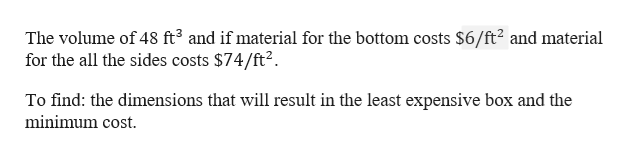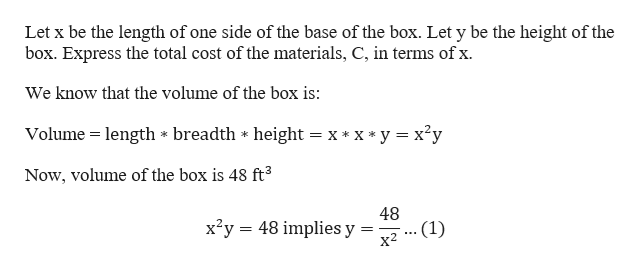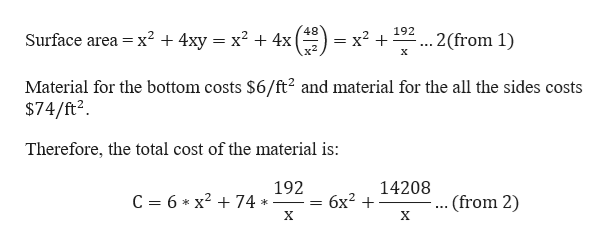# A worker is to construct an open rectangular box with a square base and a volume of 48 ft3. If material for the bottom costs​ \$6/ft2 and material for the sides costs ​\$74/ft2 what dimensions will result in the least expensive​ box? What is the minimum​ cost?Let x be the length of one side of the base of the box. Express the total cost of the​ materials, C, in terms of x.

Question

A worker is to construct an open rectangular box with a square base and a volume of 48 ft3. If material for the bottom costs​ \$6/ftand material for the sides costs ​\$74/ftwhat dimensions will result in the least expensive​ box? What is the minimum​ cost?

Let x be the length of one side of the base of the box. Express the total cost of the​ materials, C, in terms of x.

check_circle

Step 1

Note: Please check the material cost for side. Because the dimension seems to be unrealistic since 74 is a large number.

Given that a worker is to construct an open rectangular box with a square base.help_outlineImage TranscriptioncloseThe volume of 48 ft3 and if material for the bottom costs \$6/ft2 and material for the all the sides costs \$74/ft2 To find: the dimensions that will result in the least expensive box and the minimum cost fullscreen
Step 2

To begin with,help_outlineImage TranscriptioncloseLet x be the length of one side of the base of the box. Let y be the height of the box. Express the total cost of the materials, C, in terms of x. We know that the volume of the box is: Volume height = x* x*y x2y length * breadth Now, volume of the box is 48 ft3 48 x2y 48 implies y .(1) х2 fullscreen
Step 3

Since, the box is an open rectangle, the surface area of the...help_outlineImage Transcriptionclose48 = x+ x2 192 Surface area x2 + 4xy = x2 + 4x .2(from 1) х Material for the bottom costs \$6/ft2 and material for the all the sides costs \$74/ft2 Therefore, the total cost of the material is: 192 14208 C 6 x2 74 бх? + .. (from 2) X X fullscreen

### Want to see the full answer?

See Solution

#### Want to see this answer and more?

Solutions are written by subject experts who are available 24/7. Questions are typically answered within 1 hour.*

See Solution
*Response times may vary by subject and question.
Tagged in

### Functions